# 从控制理论的根轨迹法和稳定性分析谈到舵机PD控制代码实现

1.P项对系统性能影响的分析num=;
kp=1;
den=[1 1 -2];
sys=tf(num,den);
nyquist(sys);num=;
kp=10;
den=[1 1 -2];
sys=tf(num,den);
nyquist(sys);λ1和λ2为闭环传递函数的两个极点，如果λ1和λ2实部都小于0那么系统稳定，如果有虚部存在，那么就会产生振荡。由韦达定理可以求得两个极点为：num=;
kp=2.2;
den=[1 1 -2];
sys=tf(num,den);
bode(sys);


D项对系统性能的影响分析：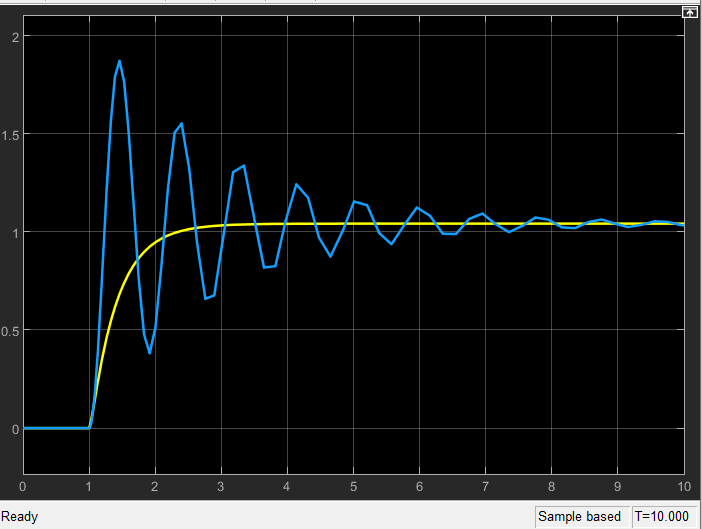1.怎么从数学角度来证明微分项加入后对系统性能的影响；
2.为什么要使用超前补偿器，他对系统会带来什么影响，为什么超前补偿器噪声不敏感。

num=;
%kp=50;
%kd=20;
den=[1 1 -2];
sys=tf(num,den);
%bode(sys);
rlocus(sys);%求根轨迹
axis([-2.5 2 -6 6]);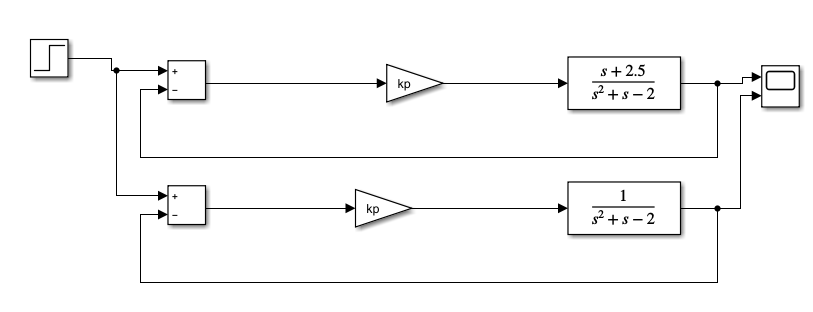num=[1 2.5];
%kp=50;
%kd=20;
den=[1 1 -2];
sys=tf(num,den);
%bode(sys);
rlocus(sys);
axis([-2.5 2 -6 6]);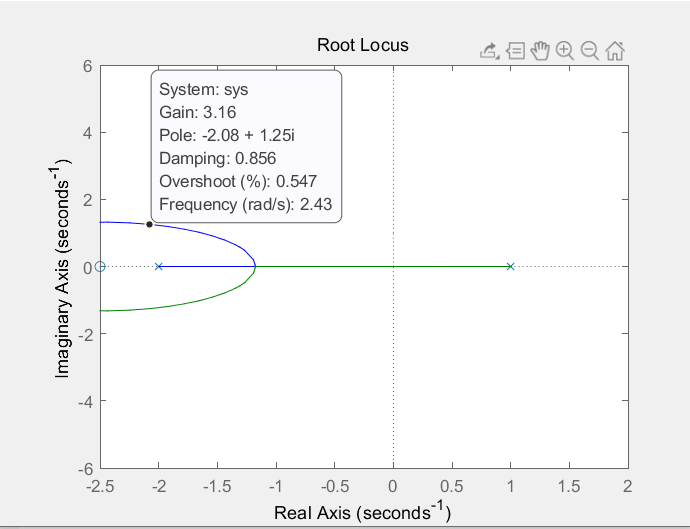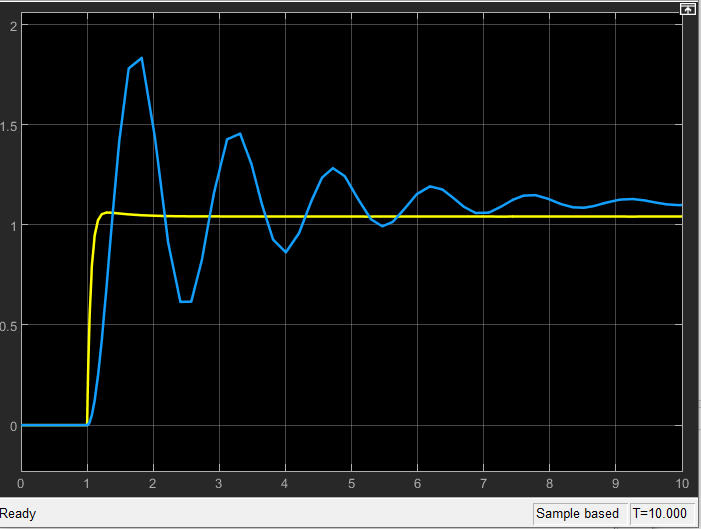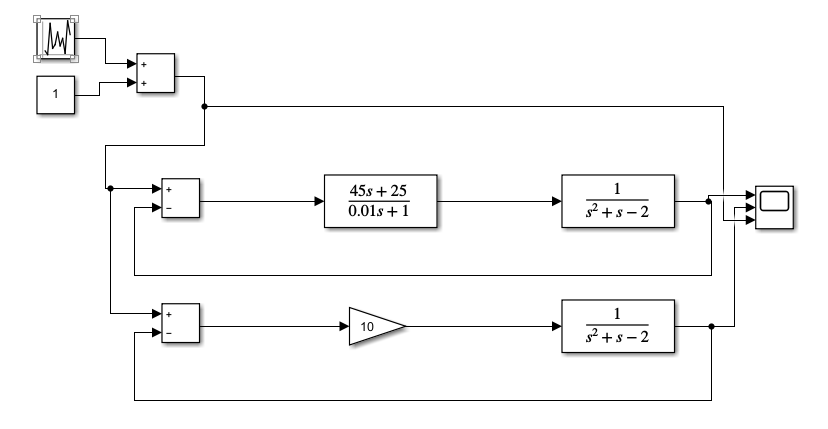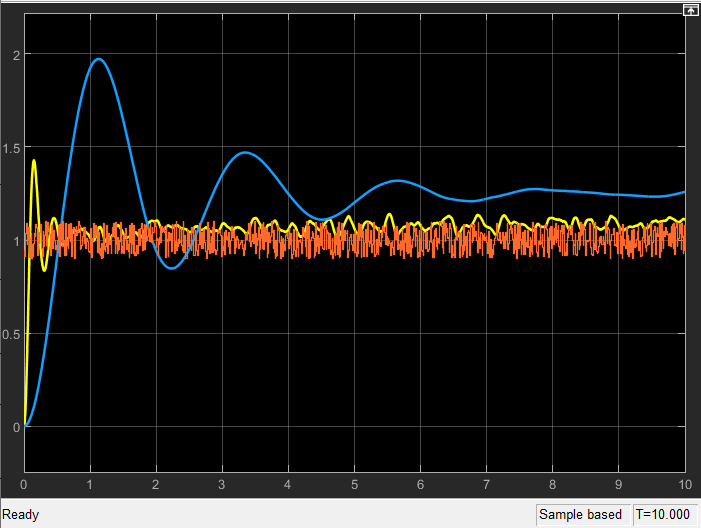PD控制C代码实现：

/*舵机的控制方法二：固定参数的舵机Pd控制*/
/*函数目的：获取控制舵机的PWM设定值*/
/*输入参数：中线平均偏差*/
/*输出参数:舵机PWM的设定值*/
int servopdcn2(float cha)
{
float nr,kp=500/90.0f;
float kd=1.2;
int PDOUT;
static int pr;
nr=cha;
PDOUT=(int)(kp*nr+kd*(nr-pr));
pr=nr;
if(PDOUT>250)
PDOUT=250;
else if(PDOUT<-250)
PDOUT=-250;
return(PDOUT);
}©️2020 CSDN 皮肤主题: 深蓝海洋 设计师: CSDN官方博客点击重新获取扫码支付1.余额是钱包充值的虚拟货币，按照1:1的比例进行支付金额的抵扣。
2.余额无法直接购买下载，可以购买VIP、C币套餐、付费专栏及课程。余额充值